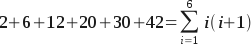SEARCH HOMEMath Central Quandaries & QueriesQuestion from fredy, a student: what is the sigma notation for the series 2+6+12+20+30+42?Hi Fredy.

You need to look at the sequence 2, 6, 12, 20, 30, 42 and figure out an algebraic pattern for the i'th term.

Two ways of doing this. One is intuitive (just playing with numbers in your head and "seeing" the answer). I think I see it.
The first number is 1 x 2, the next number is 2 x 3, the next number is 3 x 4, etc.

But if I didn't "see" this, how could I work it out mathematically? I'll show you how:

The first differences (differences between adjacent terms) are 4, 6, 8, 10, 12.
The second differences (differences between adjacent first differences) are 2, 2, 2, 2.
When the second differences are constant, you have a quadratic relationship, so you should be looking for something of the form
ti = Ai2 + Bi + C.

The zeroth term, if we project backwards, would be 2 less than the first term. That's a zero. So t0 = 0.

Thus
0 = A(0)2 + B(0) + C
C = 0.

So that simplifies it to just ti = Ai2 + Bi, which factors to i(Ai + B).

That means we can write out the terms in order
i = 1; t1 = 1(1A + B) = 2. This means 1A + B = 2.
i = 2; t2 = 2(2A + B) = 6. This means 2A + B = 3.
i = 3; t3 = 3(3A + B) = 12. This means 3A + B = 4.
i = 4; t4 = 4(4A + B) = 20. This means 4A + B = 5.

We're getting closer....
It looks like A = 1 and B = 1. Let's check if that works for the next two. 5(1) + 1 = 6. 6 x 5 = 30. Yes, that's the next term! and 6(1) + 1 = 7. 7 x 6 = 42. Yes.

So the equation we have is this:

ti = 1i2 + 1B + 0.

In factored form, that is i(i + 1). This is the same as what I saw "intuitively" above, but it is mathematically proven.

In sigma notation, you just need to know now what i to start with (since you want 2 for the first term, that is 1 x 2, so i = 1) and what i to end on (last number is 42, so that's 6 x 7, so the last i is 6).
Now we can just simply write it out putting i = 1 below the sigma to indicate where it starts and 6 at the top to show where it ends.Hope this helps,
Stephen La Rocque.Math Central is supported by the University of Regina and The Pacific Institute for the Mathematical Sciences.